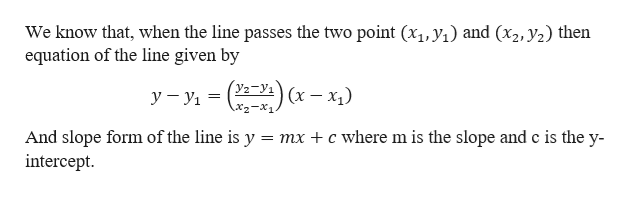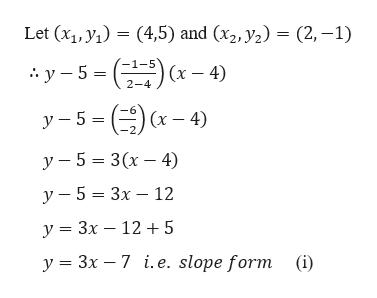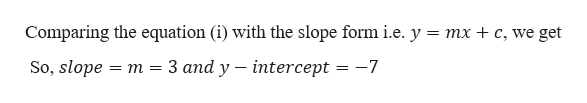# Find the equation of the line that passes through the points (4, 5) and (2, −1).Slope = ___________, y - Intercept = _______________

Question
1 views

Find the equation of the line that passes through the points (4, 5) and (2, −1).

Slope = ___________, y - Intercept = _______________

check_circle

Step 1

Given,

The line passes through the point (4,5) and (2, -1).help_outlineImage TranscriptioncloseWe know that, when the line passes the two point (x1, y) and (x2, y2) then equation of the line given by у —у, — (Уз-У:) (х — х) —х. And slope form of the line is y intercept mxc wherem is the slope and c is the y- fullscreen
Step 2

Calculationhelp_outlineImage TranscriptioncloseLet (x1, У,) — (4,5) and (xz, y2) — (2, -1) 1-5 2-4 () х у -5 — (x-4) у — 5 %3D 3(х — 4) у — 5 — 3х — 12 У %3 Зх — 12 +5 у %3 3х — 7 і.е. slope form (i) fullscreen
Step 3

Now,

...help_outlineImage TranscriptioncloseComparing the equation (i) with the slope form i.e. y mx + c, we get 3 аnd y — interсеpt %3D —7 So, slope 3 т fullscreen

### Want to see the full answer?

See Solution

#### Want to see this answer and more?

Solutions are written by subject experts who are available 24/7. Questions are typically answered within 1 hour.*

See Solution
*Response times may vary by subject and question.
Tagged in

### Other